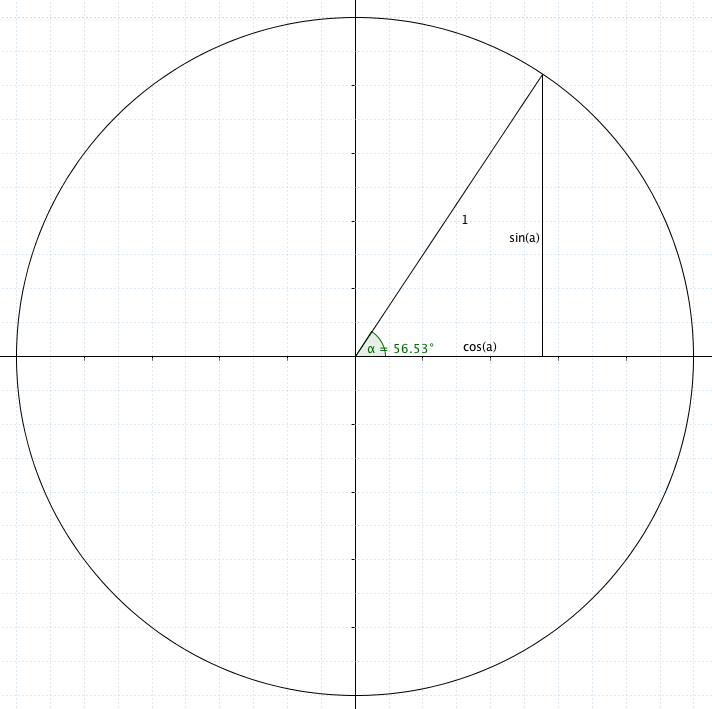Ask virtually any maths teacher what $\sec(\alpha)$ means, the chances are they’ll say “it’s $\frac{1}{\cos(\alpha)}$,” without missing a beat. Ask them what it means geometrically… well, I don’t want to speak for the teaching profession as a whole, but I’d have been stumped until the other day.

As with the bulk of geometry, it’s all to do with circles and triangles. In fact, you can ((I’d argue that you probably should)) define $\sin(\alpha)$ and $\cos(\alpha)$ using a circle and a triangle, too, which is where I’ll start ((I’m going to grit my teeth and use degrees in this post, or else I’d need to fiddle with the settings in Geogebra.)) :Draw a radius on a unit circle anywhere. From the point where the radius meets the circle, draw a vertical line to the $x$-axis, and complete the triangle by going horizontally back to the origin. Hooray, triangle. It’s got an angle at the centre, and - if you remember your GCSE trig - you can say that the opposite side (the $y$-co-ordinate of the point on the edge of the circle) is $1\times \sin (\alpha)$, while the horizontal distance (the $x$-component) is $1 \times \cos(\alpha)$.

Rather neatly, Pythagoras ((Who didn’t discover Pythagoras’ Theorem)) now tells us that $\cos^2(\alpha) + \sin^2(\alpha) = 1$.

### But what about $\sec(\alpha)$ and all those other things?

Well, the secant function - $\sec(\alpha)$, as it’s usually known - comes from another triangle, and it’s easiest to explain starting from a tangent. Unsurprisingly, the tangent to a circle is closely related to the tangent function, $\tan(\alpha)$.

If you take the same circle and radius you had before, and draw a tangent to the circle there, like this:the distance along the tangent from your key point (the one I’ve marked H) to the $x$-axis (at J) is $\tan(\alpha)$. Why? Well, again, it’s GCSE trig, plus a circle theorem: the black, red and orange lines form a triangle, and the angle at H is a right angle. (There’s the circle theorem!) You know $\tan(\alpha)$ is the opposite side to $\alpha$ divided by the adjacent side - and the adjacent side is 1! That means the opposite side is $\tan(\alpha)$.

The secant is defined as the distance along the $x$-axis from the centre of the circle to where the tangent meets - and again, you can ask Pythagoras to check that $\tan^2(\alpha) + 1 = \sec^2(\alpha)$.

### And $\cosec(\alpha)$?

It’s very similar - only on the other side of the angle! The distance from H to the $y$-axis is the cotangent ($\cot(\alpha)$) and the distance along the $y$-axis is the cosecant ($\cosec(\alpha)$). Once more, Pythagoras confirms the last of the minor trig identities.

This also suggest something about the prefix co-: you see it in cosine, cosecant and cotangent, and in all three cases it means “of the complementary angle” - $\cos(\alpha)º \equiv \sin(90-\alpha)º$, for example - the cosine of alpha is the sine of its complementary angle. The same goes for cosecant (the secant of the complementary angle) and cotangent (the tangent of the complementary angle).

Think this is useless? Ask the people who tripped up in the great C3 debacle of 2013 by not spotting that $\cos(50)º$ and $\sin(40)º$ were the same value.